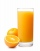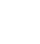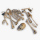# Fraction calculator

The calculator performs basic and advanced operations with fractions, expressions with fractions combined with integers, decimals, and mixed numbers. It also shows detailed step-by-step information about the fraction calculation procedure. Solve problems with two, three, or more fractions and numbers in one expression.

## Result:

### 93/5 - 77/15 = 32/15 = 2 2/15 ≅ 2.1333333

Spelled result in words is thirty-two fifteenths (or two and two fifteenths).

### How do you solve fractions step by step?

1. Conversion a mixed number 9 3/5 to a improper fraction: 9 3/5 = 9 3/5 = 9 · 5 + 3/5 = 45 + 3/5 = 48/5

To find a new numerator:
a) Multiply the whole number 9 by the denominator 5. Whole number 9 equally 9 * 5/5 = 45/5
b) Add the answer from previous step 45 to the numerator 3. New numerator is 45 + 3 = 48
c) Write a previous answer (new numerator 48) over the denominator 5.

Nine and three fifths is forty-eight fifths
2. Conversion a mixed number 7 7/15 to a improper fraction: 7 7/15 = 7 7/15 = 7 · 15 + 7/15 = 105 + 7/15 = 112/15

To find a new numerator:
a) Multiply the whole number 7 by the denominator 15. Whole number 7 equally 7 * 15/15 = 105/15
b) Add the answer from previous step 105 to the numerator 7. New numerator is 105 + 7 = 112
c) Write a previous answer (new numerator 112) over the denominator 15.

Seven and seven fifteenths is one hundred twelve fifteenths
3. Subtract: 48/5 - 112/15 = 48 · 3/5 · 3 - 112/15 = 144/15 - 112/15 = 144 - 112/15 = 32/15
For adding, subtracting, and comparing fractions, it is suitable to adjust both fractions to a common (equal, identical) denominator. The common denominator you can calculate as the least common multiple of both denominators - LCM(5, 15) = 15. In practice, it is enough to find the common denominator (not necessarily the lowest) by multiplying the denominators: 5 × 15 = 75. In the following intermediate step, it cannot further simplify the fraction result by canceling.
In other words - forty-eight fifths minus one hundred twelve fifteenths = thirty-two fifteenths.

#### Rules for expressions with fractions:

Fractions - simply use a forward slash between the numerator and denominator, i.e., for five-hundredths, enter 5/100. If you are using mixed numbers, be sure to leave a single space between the whole and fraction part.
The slash separates the numerator (number above a fraction line) and denominator (number below).

Mixed numerals (mixed fractions or mixed numbers) write as integer separated by one space and fraction i.e., 1 2/3 (having the same sign). An example of a negative mixed fraction: -5 1/2.
Because slash is both signs for fraction line and division, we recommended use colon (:) as the operator of division fractions i.e., 1/2 : 3.

Decimals (decimal numbers) enter with a decimal point . and they are automatically converted to fractions - i.e. 1.45.

The colon : and slash / is the symbol of division. Can be used to divide mixed numbers 1 2/3 : 4 3/8 or can be used for write complex fractions i.e. 1/2 : 1/3.
An asterisk * or × is the symbol for multiplication.
Plus + is addition, minus sign - is subtraction and ()[] is mathematical parentheses.
The exponentiation/power symbol is ^ - for example: (7/8-4/5)^2 = (7/8-4/5)2

#### Examples:

subtracting fractions: 2/3 - 1/2
multiplying fractions: 7/8 * 3/9
dividing Fractions: 1/2 : 3/4
exponentiation of fraction: 3/5^3
fractional exponents: 16 ^ 1/2
adding fractions and mixed numbers: 8/5 + 6 2/7
dividing integer and fraction: 5 ÷ 1/2
complex fractions: 5/8 : 2 2/3
decimal to fraction: 0.625
Fraction to Decimal: 1/4
Fraction to Percent: 1/8 %
comparing fractions: 1/4 2/3
multiplying a fraction by a whole number: 6 * 3/4
square root of a fraction: sqrt(1/16)
reducing or simplifying the fraction (simplification) - dividing the numerator and denominator of a fraction by the same non-zero number - equivalent fraction: 4/22
expression with brackets: 1/3 * (1/2 - 3 3/8)
compound fraction: 3/4 of 5/7
fractions multiple: 2/3 of 3/5
divide to find the quotient: 3/5 ÷ 2/3

The calculator follows well-known rules for order of operations. The most common mnemonics for remembering this order of operations are:
PEMDAS - Parentheses, Exponents, Multiplication, Division, Addition, Subtraction.
BEDMAS - Brackets, Exponents, Division, Multiplication, Addition, Subtraction
BODMAS - Brackets, Of or Order, Division, Multiplication, Addition, Subtraction.
GEMDAS - Grouping Symbols - brackets (){}, Exponents, Multiplication, Division, Addition, Subtraction.
Be careful, always do multiplication and division before addition and subtraction. Some operators (+ and -) and (* and /) has the same priority and then must evaluate from left to right.

## Fractions in word problems:

• KingKing had four sons. First inherit 1/2, second 1/4, third 1/5 of property. What part of the property was left to the last of the brothers?
• The recipeThe recipe they are following requires 7/8 cups of milk, Tom already put 3/8 cups of milk. How much milk should Lea add to follow the recipe?
• ShopperEva spent 1/4 in one store and 1/3 in another. What fraction is left?
• Empty and fullAn empty can has a mass of 1/6 lb. When it is filled with sand, it has a mass of 7/12 lb. Find the mass of the sand in the can?
• MichaelMichael had a bar of chocolate. He ate 1/2 of it and gave away 1/3. What fraction had he left?
• PatelPatel squeezed oranges so that his family could have fresh-squeezed juice for breakfast. He squeezed 4/17 cups from the first orange, 3/10 cups from the second orange, StartFraction 9 over 20 E
• Pizza cover5/8 of the pizza is covered in mushrooms, 1/4 of the pizza is covered with pineapples, and the rest of the pizza is covered with only cheese. What fraction of the pizza is covered with only cheese?
• SchoolThere are 150 pupils in grade 5 . 2/3 of them are female. By what fractions are the males?
• SarahSarah has a photography blog. 3/7 of her photos are of nature, 1/4 of the rest are of her friends. What fraction of all of Sarah's photos is of her friends?
• Cups of flourJade was baking cupcakes for her class. She has 4 5/4 cups of flour.  For one batch, she used 1 2/3 cups of flour.  On another batch, she used 7/9 of a cup of flour.  How much flour does Jade have left after making the two batches of cupcakes?
• Farmer PeterFarmer Peter paints 12 chicken coop. He started painting this day morning. Now he only has 1/4 of the chicken coop left to paint this afternoon. How many chicken coops did farmer Peter paint this morning?
• Difference mixed fractionsWhat is the difference between 4 2/3 and 3 1/6?
• Human 3Human bones are 3/10 living tissues 9/20 minerals, and the rest water. What fraction of human bone is water?